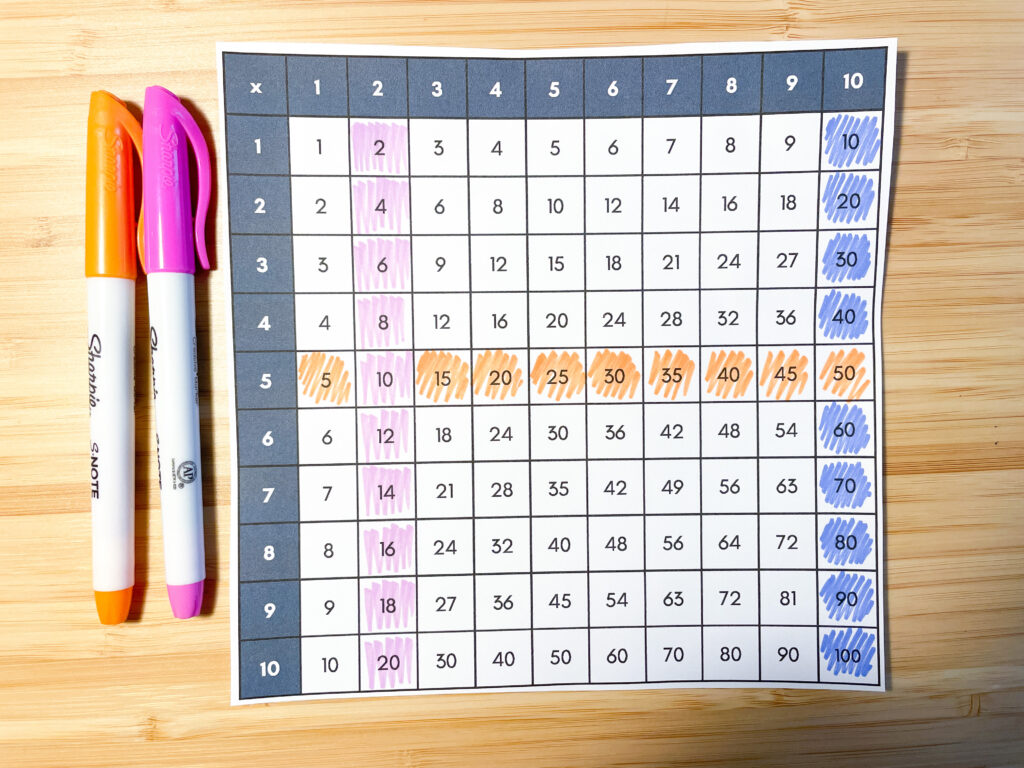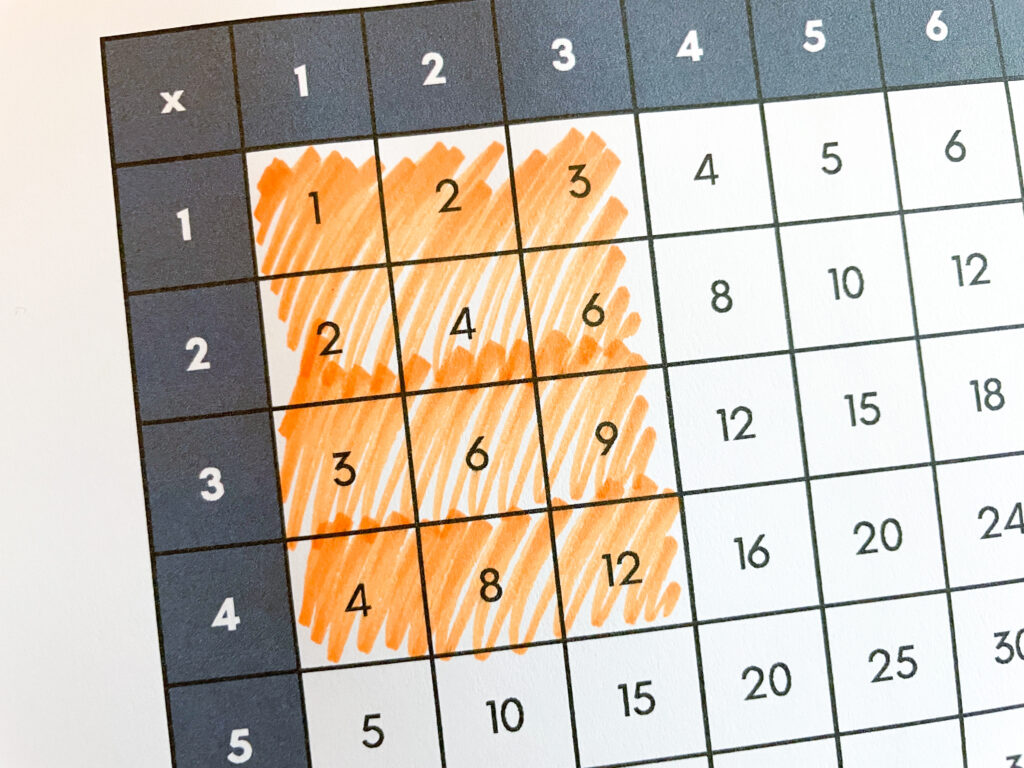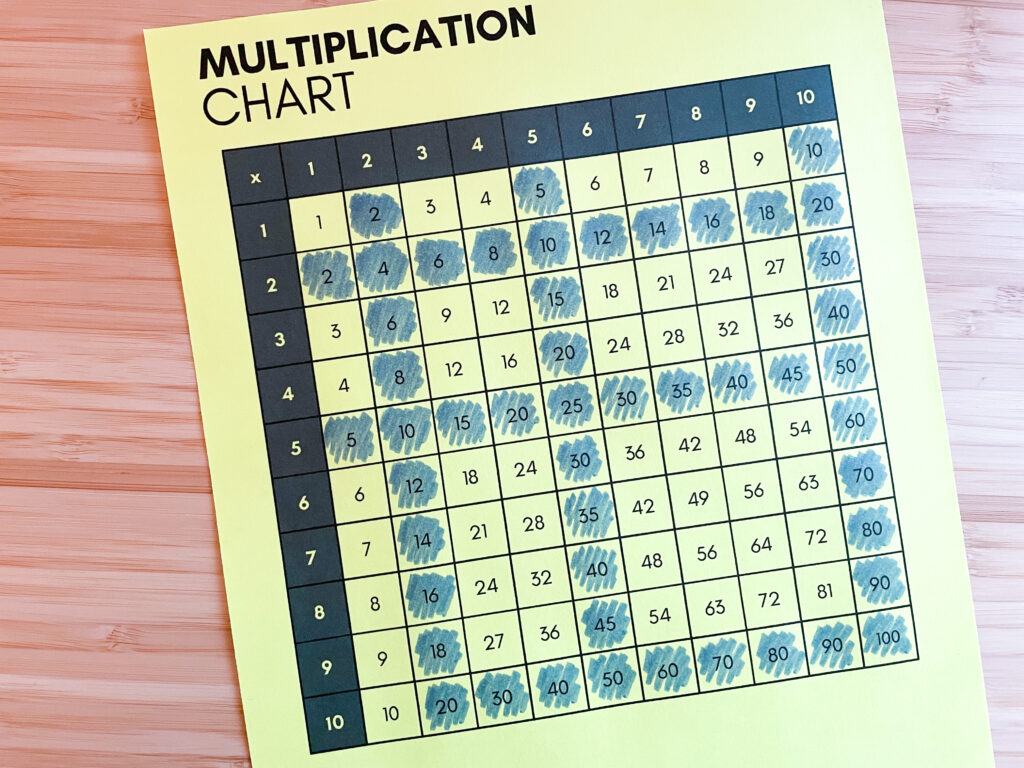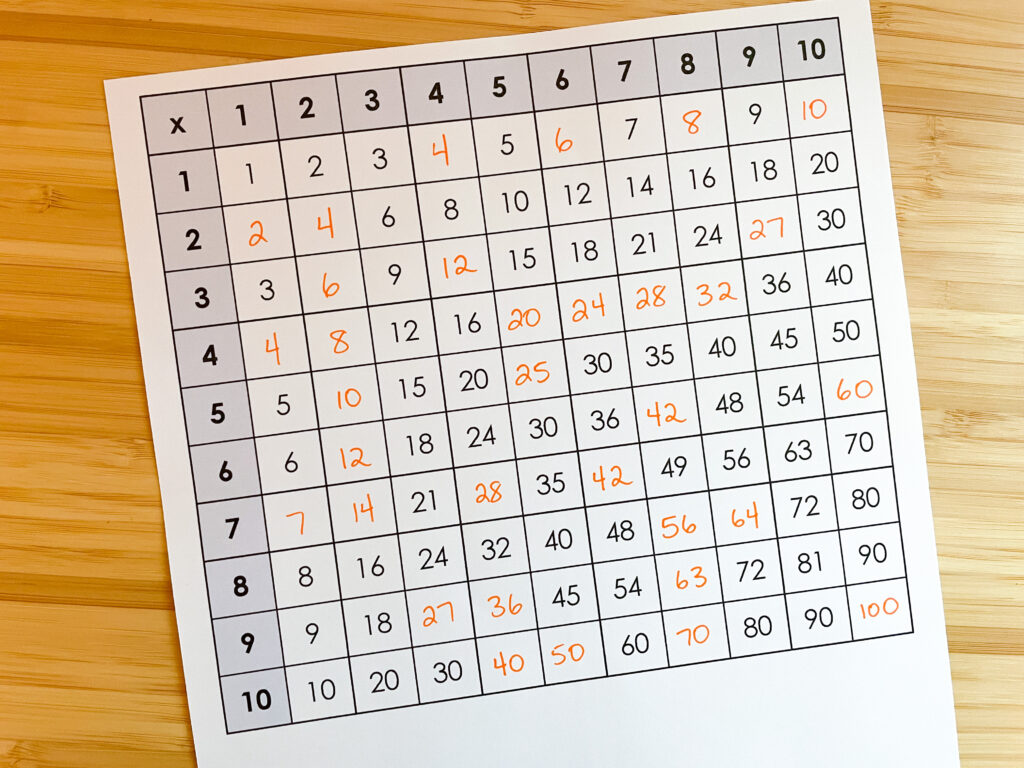# 5 Fun Ways to Use a Multiplication ChartMultiplication charts are a staple in many Grades 3-6 classrooms. But how can you use this important tool in a fun way to reinforce multiplication facts? Multiplication charts are more than just a reference tool to find answers to problems – they can support students in a fun way as they learn and practice the times tables!

Here are five fun ways to use a multiplication chart in your elementary classroom. (And be sure to download your free multiplication chart printables down below!)

## Cut it up to create a puzzle.

Cut the chart into about ten different pieces and have students put it back together at a math center. To do this, students will need to find products and understand how it works.## Find patterns.

Multiplication charts include lots of different patterns. In the different rows and columns we see skip-counting patterns that we can use when we solve multiplication problems. Have students notice the patterns and make connections to the multiplication facts. For example, in the 5’s row we skip-count by 5’s. We can use that skip counting sequence to solve a problem like 4×5 by skip counting by 5’s four times.## Use the multiplication chart to create arrays.

We can create an array and relate it to an array created with physical objects. Here we see a four by three array. As long as we start the array in the top left corner, the product will be shown in the bottom right. Remember that the more ways we see something, the better!## Times Tables Posters

Bright classroom posters for the multiplication facts

## Shade known facts on the multiplication chart.

The number of multiplication facts to learn can be overwhelming. But it becomes a lot less overwhelming when students shade the ones they already know. The chart below shows what this might look like once a student has learned the x2, x10, and x5 facts. The number of facts left to learn decreases rapidly (because of the commutative property). When students get to the more difficult facts, there are not many left to learn at all! A mutiplication chart can serve as a good visual for this. I wrote a post about this in more detail which you can view here.## Fill in the missing numbers.

Have your students fill in missing numbers on a multiplication chart to reinforce their knowledge of multiplication patterns.## Free Multiplication Chart

If you’d like to try some of these activities with your students, here are some free multiplication chart printables you can download and use!

This site uses Akismet to reduce spam. Learn how your comment data is processed.